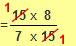## The Four Operations for Fractions

### Dividing Fractions

This is virtually a rearranging of multiplying fractions.

The basic steps to follow are:

1. Change all numbers into improper fractions.
2. Keep the 1st fraction as it is and change the ‘÷’ sign into a ‘x’ sign.
3. Flip the 2nd fraction.
4. Now carry out the multiplying, cancelling anything you can, as you go along.
5. Simplify.

## Examples

Example 1

 2 ÷ 3 5

 = 2 ÷ 3 1 5
(Step 1)

 = 2 x 5 1 3
(Steps 2 & 3)

 = 10 3
(Step 4)

 = 3 1 3
(Step 5)

Example 2

 2 1 ÷ 1 7 7 8

 = 15 ÷ 15 7 8
(Step 1)

 = 15 x 8 7 15
(Step 2 & 3)(Step 4)

 = 1 x 8 7 x 1

 = 8 7

 = 1 1 7
(Step 5)